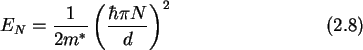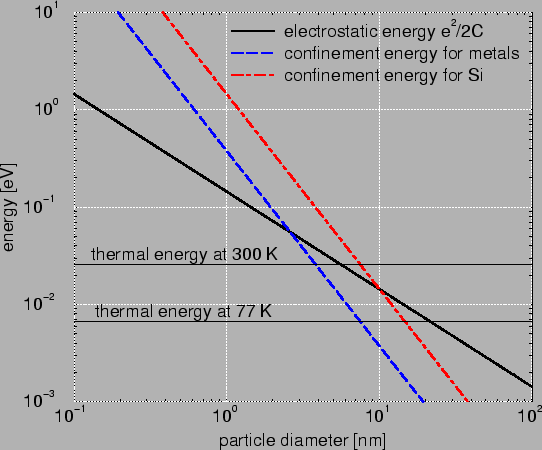Next: 2.2 Work Done by Up: 2.1 Characteristic Energies Previous: 2.1.1 Electron Electron Interaction

## 2.1.2 Quantum Confinement Energies

With decreasing island size the energy level spacing of electron states increases indirectly proportional to the square of the dot size. Taking an   infinite potential well as a simple model for a quantum dot, one calculates by solving Schrödinger's equation (see Appendix C.1) the  energy levels toBecause of the lower  effective mass in Si compared to Al, the quantum confinement energy is bigger in Si (see Fig. 2.3).For very small particle diameters (around 1 nm) the formulas (2.6), (2.7), and (2.8) cease to be valid. The concept of the effective mass is based on a periodic lattice and starts to break down for small islands. One would have to apply cluster theory to calculate the energy levels more precisely. In reality slightly smaller confinement energies than predicted in (2.8) are observed, which may partly be attributed to the model of an infinite well. The energy levels slightly decrease in the case of a finite well (see Appendix C.2). Furthermore, the lattice constant for metals is about 0.4 nm whereas for semiconductors it is almost 0.6 nm. Thus the concept of carrier densities is not valid anymore.

Room temperature operation is achievable with structures smaller than 10 nm. Cooling single-electron devices with liquid nitrogen reduces thermal fluctuations to some extent, but relaxes the size constrains only by a factor of two.Next: 2.2 Work Done by Up: 2.1 Characteristic Energies Previous: 2.1.1 Electron Electron Interaction

Christoph Wasshuber